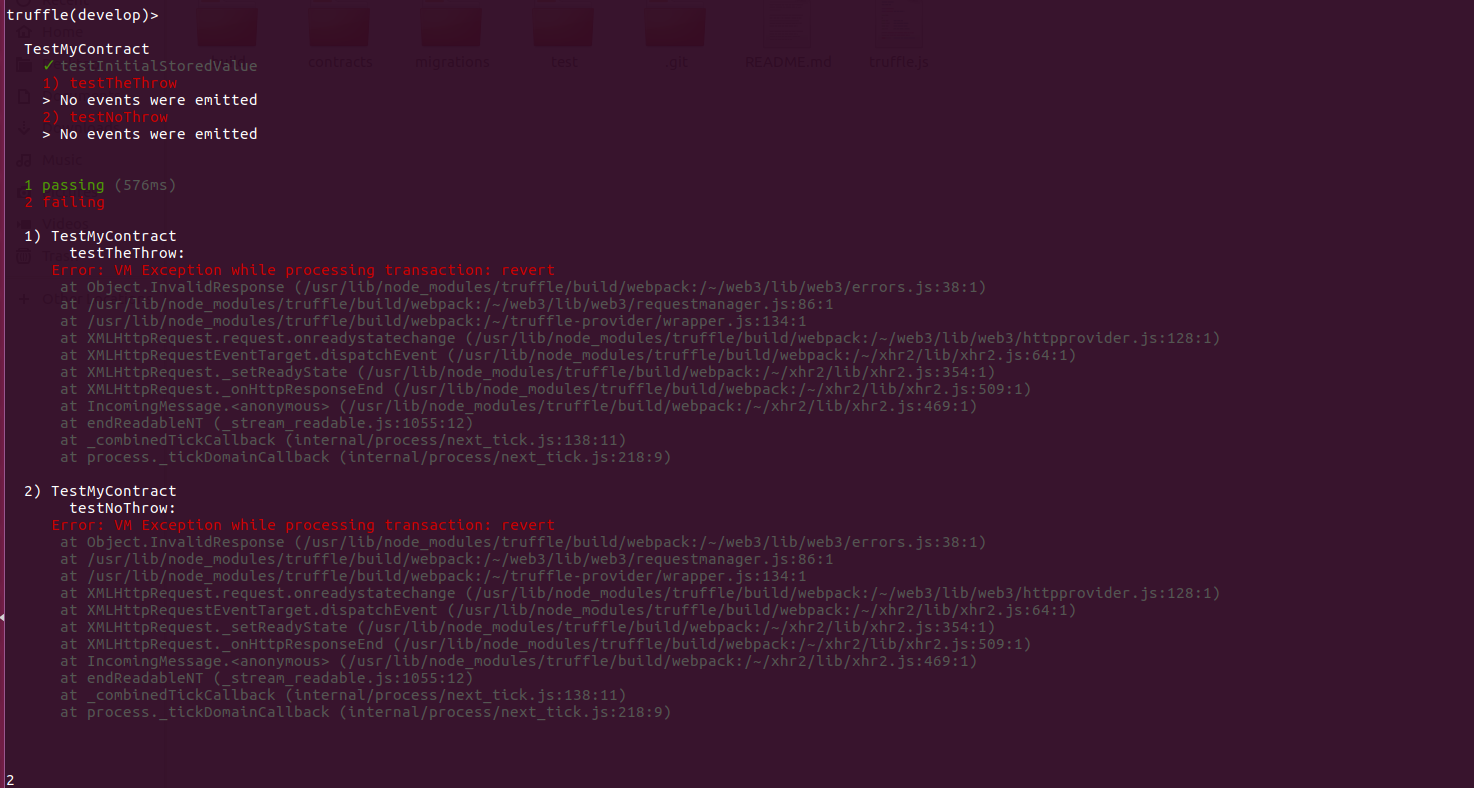# Transaction reverted when testing requires/asserts in non void functions

im trying to test the requires of a Smart Contract using just solidity according to this article:

http://truffleframework.com/tutorials/testing-for-throws-in-solidity-tests

This is the contract, the throw proxy contract and the test:

``````/* Testing with solidity tests. */

import "truffle/Assert.sol";
import "../contracts/MyContract.sol";

contract TestMyContract {

function testInitialStoredValue() {
MyContract mycontract = new MyContract();

uint expected = 24;

Assert.equal(mycontract.mynumber(), expected, "First number set should be 24.");
}

function testTheThrow() {
MyContract mycontract = new MyContract();

uint num = 7;

bool r = throwproxy.execute.gas(200000)();

Assert.isFalse(r, "Should be false because is should throw!");

}

function testNoThrow() {
MyContract mycontract = new MyContract();

bool r = throwproxy.execute.gas(200000)();

Assert.isTrue(r, "Should be true because is should throw!");

}

}

// Proxy contract for testing throws
contract ThrowProxy {
bytes data;

target = _target;
}

//prime the data using the fallback function.
function() {
data = msg.data;
}

function execute() returns (bool) {
return target.call(data);
}
}
``````

If i run the tests i get this error:If i change the storeNum function to void from

`````` function storeNum(uint mynum)
public
returns (bool success)
{
require(mynum > 10);
mynumber = mynum;
return true;
}
``````

to

`````` function storeNum(uint mynum)
public
{
require(mynum > 10);
mynumber = mynum;
return true;
}
``````

the tests works..

Any ideas?

Im using Truffle v4.1.11

EDIT:

I have submited an issue in truffle github, maybe the recent changes on solidity has this constraint or breaks the test using a proxy

https://github.com/trufflesuite/truffle/issues/1001

`````` function storeNum(uint mynum)
public
returns (bool success)
{
require(mynum > 10);
mynumber = mynum;
return true;
}
``````

Try removing `success` as a return variable since you are not returning that specific variable:

`````` function storeNum(uint mynum)
public
returns (bool)
{
require(mynum > 10);
mynumber = mynum;
return true;
}
``````

If you wanted to keep `returns (bool success)` you could do something like this:

`````` function storeNum(uint mynum)
public
returns (bool success)
{
require(mynum > 10);
mynumber = mynum;
success = true;
}
``````
• Hi, i understand, but that isnt seems to be the cause of the problem, im getting the same error. Not only with boolean values, also with numeric. I have submited an issue into truffle an edited the question – Marcos Martínez Jun 12 '18 at 16:56